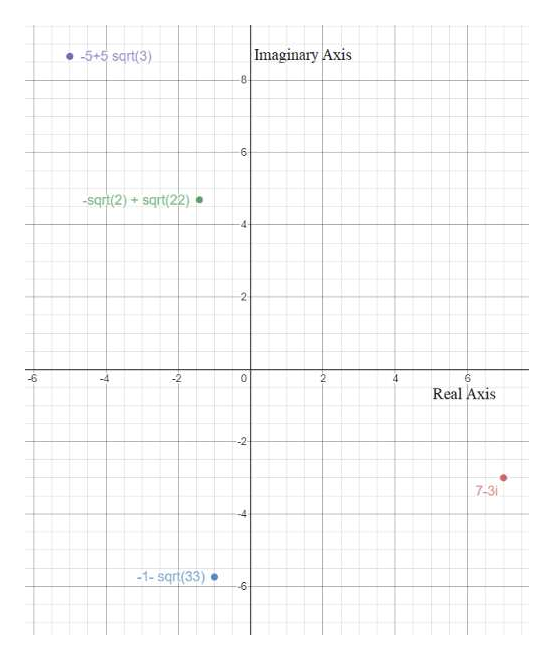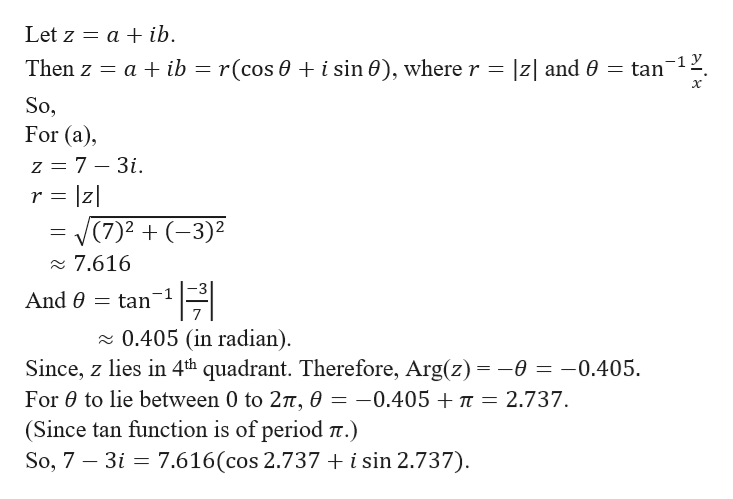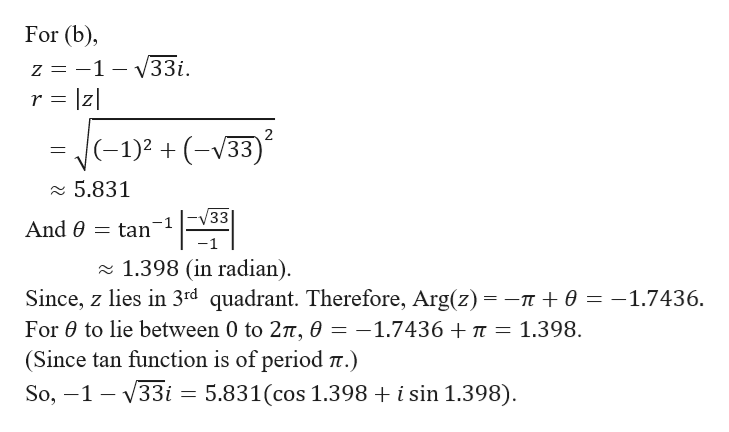# Graph the complex number, find its modulus and put the complex number in polar form with argument θ between 0 and 2π (a) 7−3i(b) −1−√33i(c) −√2+i√22(d) −5 + 5√3i

Question
1 views
Graph the complex number, find its modulus and put the complex number in polar form with argument θ between 0 and 2π

(a) 7−3i
(b) −1−√33i
(c) −√2+i√22
(d) −5 + 5√3i
check_circle

Step 1

First, we will graph the given complex numbers.

(a) 7−3i

(b) −1−√33i

(c) −√2+i√22

(d) −5 + 5√3ihelp_outlineImage TranscriptioncloseImaginary Axis 5+5 sqrt(3) 8- 6 sqrt(2)+sqrt(22) 4 2 -6 0 4 6 Real Axis -2 7-3 -1-sqrt(33) -6 fullscreen
Step 2

Let us first know the method to convert a complex number to its polar form.help_outlineImage TranscriptioncloseLet z aib = a ib r(cos 0 i sin0), where r = Then z z and e tan 1 х So, For (a) z 7 - 3i r lz V(7)23)2 2 7.616 And tan 0.405 (in radian). Since, z lies in 4th quadrant. Therefore, Arg(z)= -0 = -0.405 For e to lie between 0 to 27t, 0 (Since tan function is of period t.) So, 7 3i 7.616(cos 2.737 i sin 2.737) -0.405T 2.737. fullscreen
Step 3

Similarly,   ...help_outlineImage TranscriptioncloseFor (b) -1- V33i r z = Z 2 (-1)2(V33 5.831 /33 And 0 = tan 1.398 (in radian) Since, z lies in 3rd quadrant. Therefore, Arg(z) = -n + 0 = -1.7436. For to lie between 0 to 27t, e = -1.7436 +7T (Since tan function is of period 7t.) 1.398 So, 1 33i = 5.831(cos 1.398 + i sin 1.398) fullscreen

### Want to see the full answer?

See Solution

#### Want to see this answer and more?

Solutions are written by subject experts who are available 24/7. Questions are typically answered within 1 hour.*

See Solution
*Response times may vary by subject and question.
Tagged in

### Algebra